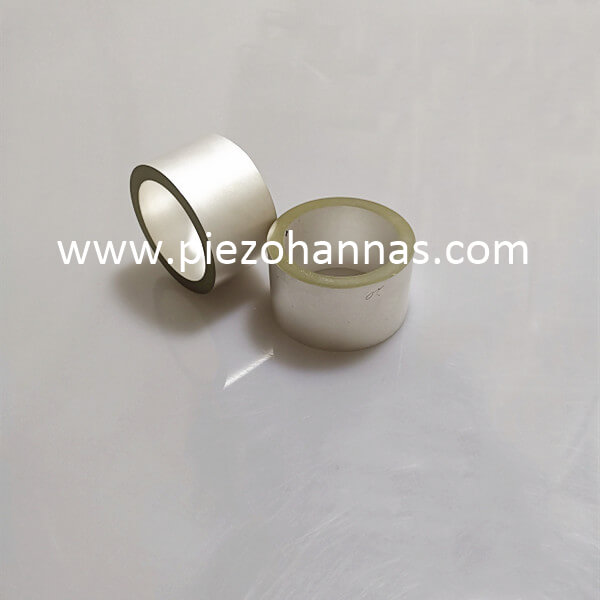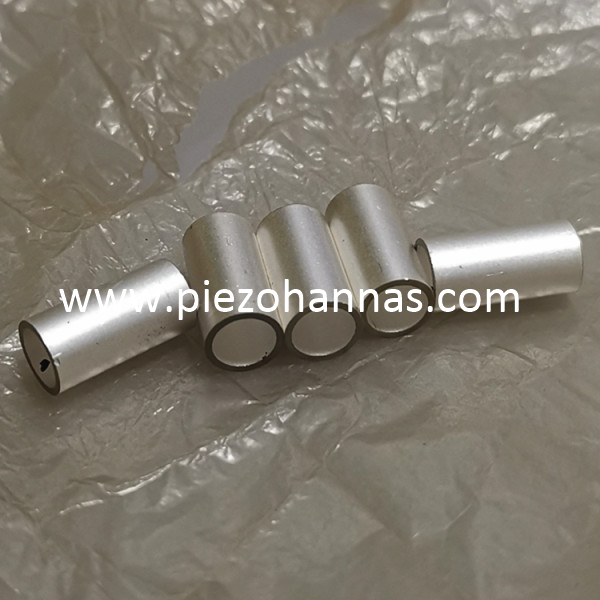Piezo Hannas (WuHan) Tech Co,.Ltd.－Professional Piezoceramic Elements Supplier
• Product Name
• Product Keyword
• Product Model
• Product Summary
• Product Description
• Multi Field Search
News
You are here: / / / Dynamics Research Of Piezoelectricity Tube

Dynamics Research Of Piezoelectricity Tube

Views:0     Author:Site Editor     Publish Time: 2019-09-19      Origin:Site

Piezoelectric ceramics are a type of crystal material that undergoes a shape change such as compression or elongation when subjected to mechanical stress. As the shape changes, different charges are generated on both sides of the crystal. Conversely, adding a different voltage across the linear piezo tubes will result in a corresponding mechanical displacement or stress. The quarter-piezoelectric ceramic tube is a hollow cylindrical ceramic tube with a conductive coating on the inner cylindrical surface and four conductive layers that are equal in area but insulated from each other. Applying a voltage between the conductive layer of the inner surface and the conductive layer of the outer surface can cause the ceramic tube to produce a form of motion such as bending, elongation, or a spherical crown. In order to properly use ceramic tubes, dynamic analysis must be performed.

Dynamic Analysis of Ceramic Tubes in ElongationAssuming that the applied voltage is zero voltage applied to the tube, the four electrodes outside the tube are simultaneously applied with a positive voltage in the same direction. Then there is £1:1 S12· + d31·E3 where £ is strain, and numbers 1 to 6 represent coordinate vector directions, which represent z, Y, yz, z, xy, respectively. Sl2 represents the modulus of elasticity, d. Indicates the length and E is the electric field strength. Where b is the viscosity coefficient; is the displacement; J0 is the ceramic density; A is the ceramic tube cross-sectional area; sfi is the piezoelectric coefficient.

The motion analysis of the ceramic tube when bending is analyzed from the static analysis of the human hand, and the deformation is analyzed by geometric method. In the formula, l is the length of the ceramic tube; M is the mass of the ceramic tube per unit length.

Simulation analysis

Through the above theoretical analysis, combined with the mechanical equations of elongation and bending of piezoelectric cylinder transducer, ANSYS software was used in the experimental research. The modal analysis and transient dynamics analysis of the ceramic tube are carried out in the analysis, taking into account the meshing and actual conduction when establishing the finite element model. The four-cent cylindrical cylinder is built and bonded together as a whole to ensure that the adhesion between the two cylinders is not conductive, but the force transmission and deformation conform to the real situation.

Finite element mesh division of quarter piezoelectric ceramic tube

The quarter-piezoelectric ceramic tube is a typical electromechanical coupling device. Therefore, for the grid division unit of the quarter-piezoelectric ceramic tube, the finite element type is supporting the coupled field analysis must be used. Coupled field analysis is supported in the lens3, solid5, and solid98 units of ANSYS software, where solid5 can be used to analyze piezoelectric materials. The solid5 model is a three-dimensional unit consisting of eight nodes forming a hexahedral structure with a maximum of six degrees of freedom per node. Only three displacement degrees of freedom and one voltage degree of freedom are used for the analysis of piezoelectric materials. The finite piezo element diagram of the piezoelectric ceramic tube divided by the solid5 machine-electric coupling unit is shown.These include 2,160 sol-id5 units and 6,020 solid95 units. The degree of freedom of solid5 is set to four, which are the displacements UX, UZ and a voltage degree of freedom VOLT in the X, y, and Z directions respectively.

Modal analysis of quarter piezoelectric ceramic tubes

Modal analysis is the basis of dynamic transient analysis, which can determine the vibration characteristics of the quarter piezoelectric ceramic tube, namely the natural frequency and mode shape of the structure, providing a theoretical basis for the design of the drive circuit. The modal analysis of a piezoceramic tube first defines the boundary conditions with a symmetrical structure. According to the actual situation in the application process, a zero displacement constraint is applied to the bottom end surface of the electrode. At the same time, the potential boundary conditions of the piezoelectric ceramic are defined, and the upper and lower electrodes of the adjacent two piezoelectric ceramic sheets are coupled by a node, and are also defined as an electrical disconnection. The modal analysis of the system structure is then performed using the modal complete solver provided by ANSYS.

Transient analysis of quarter piezoelectric ceramic tubes

The transient dynamics analysis was performed to observe the dynamic response of the ceramic tube as it undergoes any time-varying load. In the ANSYS analysis, the sawtooth voltage was applied to the quarter piezoelectric ceramic tube to obtain the bending vibration of the piezo ceramic tube. In the analysis process, due to the correlation between load and time, inertial force and damping are two important aspects to be considered. The damping matrix is obtained by Raylwigh damping constant a, multiplied by mass matrix and stiffness matrix respectively. The electromechanical coupling unit in the finite piezo element model of ceramic tube is a nonlinear unit, which brings difficulties to the solution of transient dynamic analysis. Therefore, the time-integral method is used to solve the dynamic differential equations at discrete time points. The time increment is called the integration time step (ITS), and the size of the step directly affects the accuracy of the transient analysis solution. The step size is an important parameter in the analysis. The smaller the time step, the higher the accuracy. However, too small a time integration step will waste computing resources and may even lead to numerical analysis not being completed. If the step size is too large, the calculation error of the higher-order modal response of the quarter piezoelectric ceramic tube mechanism will be caused. Therefore, according to the actual situation, the driving waveform used in this experiment is the sawtooth wave voltage, and this voltage does not appear serious waveform distortion.At the same time, combined with the modal analysis results, in the selection of the integration time step, the main consideration is to solve the response frequency, the time step should be small enough to solve the motion response of the structure. According to the sampling theorem, the optimal time step is TS≤1/20f (the factory is the natural frequency of the quarter-piezoelectric ceramic tube, the natural frequency is obtained from the product parameter specification, and TS≤1/(10×2 242) is obtained. The ITS is taken as 1.22 S, so the integration time step of the transient analysis of the quarter piezoelectric ceramic tube is taken as 1 s. In summary, when analyzing piezoelectric vibrators, due to a variety of nonlinear factors such as large deformation, material nonlinearity and contact, the complete system matrix is used to calculate the transient dynamic response, that is, without any form of simplification of the matrix. This will minimize the effects of nonlinearity. The transient dynamics analysis and solution are performed on the quarter piezoelectric ceramic tube mechanism. The analysis results are as follows. When the sawtooth voltage applied to the quarter piezoelectric ceramic tube is 100 Hz and the amplitude is 150 V,The curve of the displacement in the y-direction of the piezoceramic tube shown as a function of time was obtained. When a step signal with a voltage amplitude of 270 V is applied, the top end of the piezoceramic tube is displaced in the y direction.

The quarter Pzt material piezoelectric tube will generate different amplitude stresses under the action of the sawtooth voltage. The stress is analyzed, and the result can give a certain guidance to the installation of the piezoelectric ceramic tube, and can predict the position where fatigue occurs.it shows the global stress of the vibrator body during transient analysis, and shows the maximum local stress. the darker the color, the greater the stress. It can be seen that the top of the ceramic tube is its stress concentration zone, where fatigue and fracture are most likely to occur, which will provide theoretical guidance for installation and application of loads.

A dynamic theoretical model of a four-point piezoceramic tube was established. Based on the relationship between the displacement and the voltage during the bending and elongation of the quarter piezoelectric ceramic tube, the dynamic equation of the piezo ceramic tube is given. The modal analysis and transient dynamics analysis of the quarter ceramic tube were carried out by the finite element analysis software ANSYS. The displacement curves driven by the sawtooth voltage and the step voltage were obtained, and the prescription of the quarter piezoelectric ceramic tube was analyzed. The distribution is verified to verify the correctness of the equation.

Feedback
Piezo Hannas (WuHan) Tech Co,.Ltd  is a professional piezoelectric ceramics and ultrasonic transducer manufacturer, dedicated to ultrasonic technology and industrial applications.